Question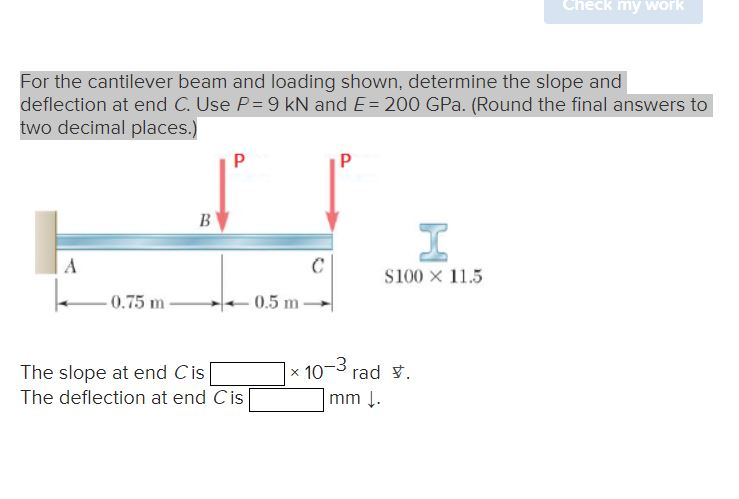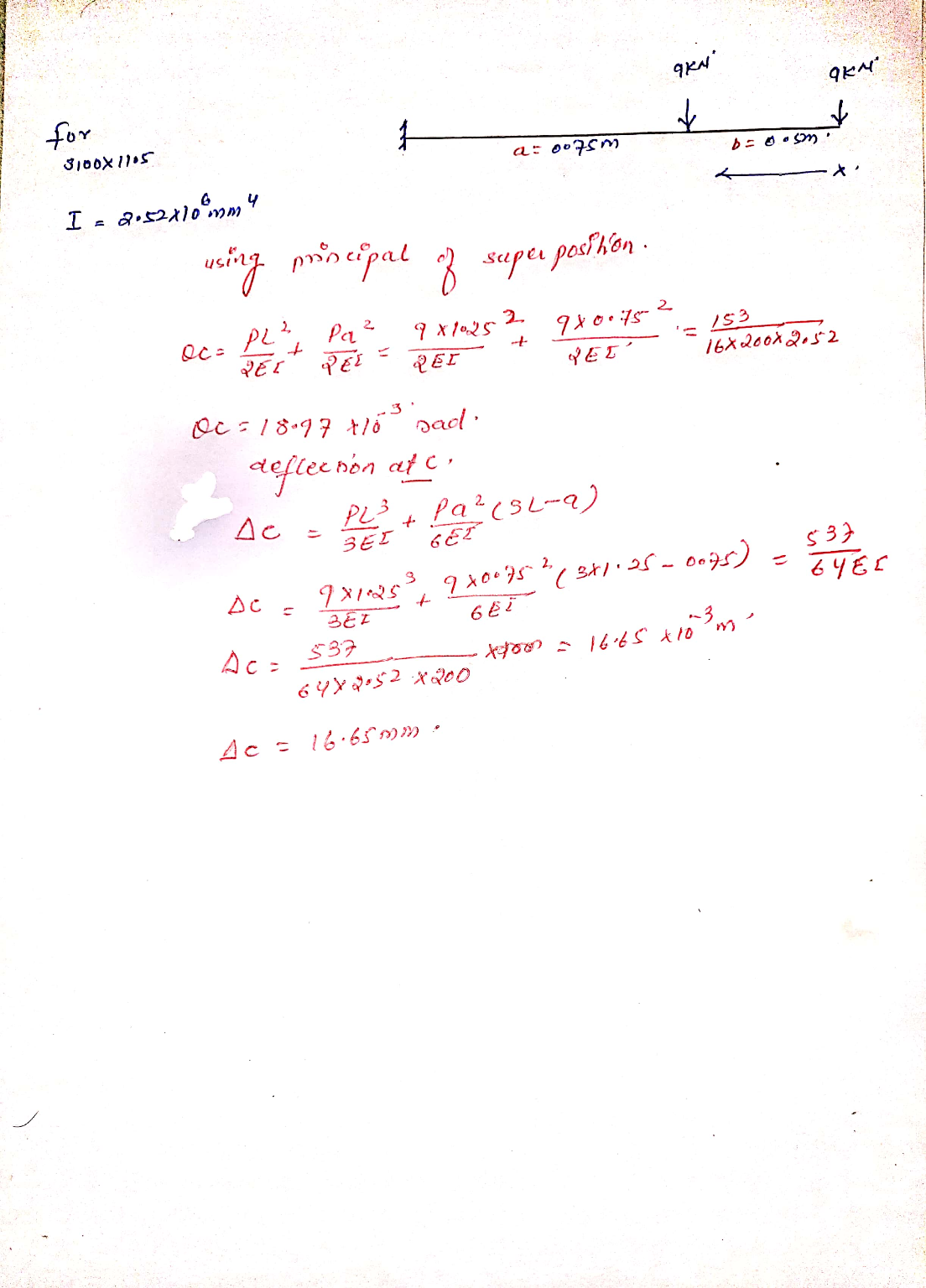#### Earn Coins

Coins can be redeemed for fabulous gifts.

Similar Homework Help Questions
• ### For the cantilever beam and loading shown, determine the slope and deflection at point B. Use...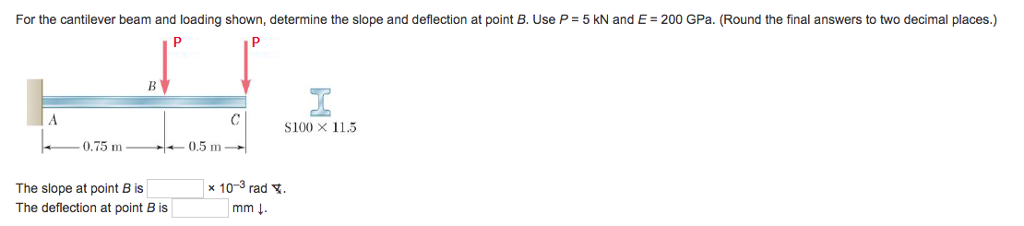For the cantilever beam and loading shown, determine the slope and deflection at point B. Use P 5 kN and E 200 GPa. (Round the final answers to two decimal places.) S100 X 11.5 0.75 m 0.5 m The slope at point B is The deflection at point B is x 10-3 rad. mm ↓

• ### Check my For the cantilever beam and loading shown, determine the slope and deflection at point...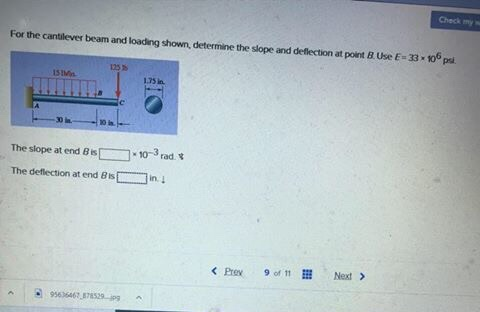Check my For the cantilever beam and loading shown, determine the slope and deflection at point Use E3310 The slope at end Bis The deflection at end BC 10-3 rad. n . < Prey 9 of 11 Next > 06446

• ### For the cantilever beam and loading shown, determine the slope and deflection at end C. Use...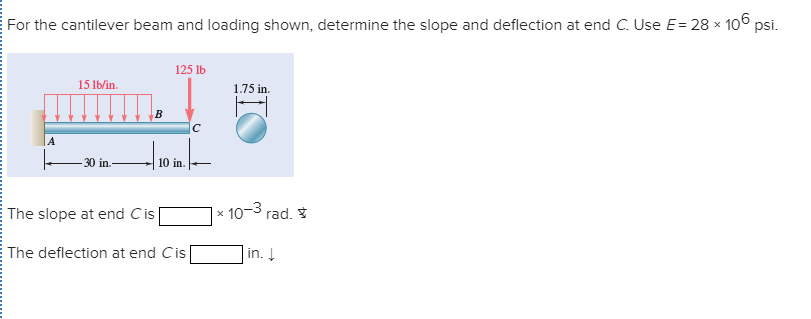For the cantilever beam and loading shown, determine the slope and deflection at end C. Use E= 28 × 106 psi. 125 lb 15 lb/in. 1.75 in. |A 30 in. 10 in. x 10-3 The slope at end Cis rad. * in. Į The deflection at end Cis

• ### Q1. For the cantilever beam and loading shown with circular section of 60 mm diameter and...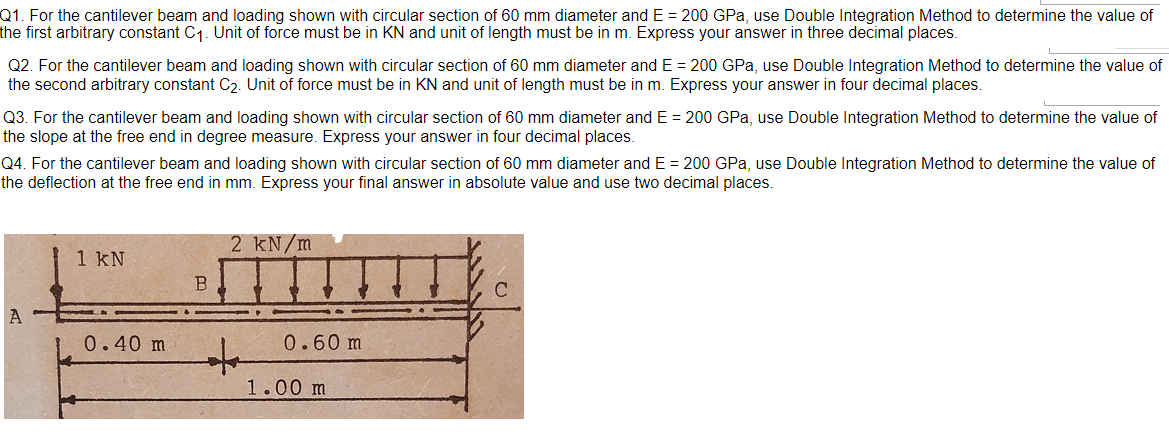Q1. For the cantilever beam and loading shown with circular section of 60 mm diameter and E = 200 GPa, use Double Integration Method to determine the value of the first arbitrary constant C1. Unit of force must be in KN and unit of length must be in m. Express your answer in three decimal places. Q2. For the cantilever beam and loading shown with circular section of 60 mm diameter and E = 200 GPa, use Double Integration Method...

• ### 4. Determine the slope and deflection at end point C of the cantilever beam shown in...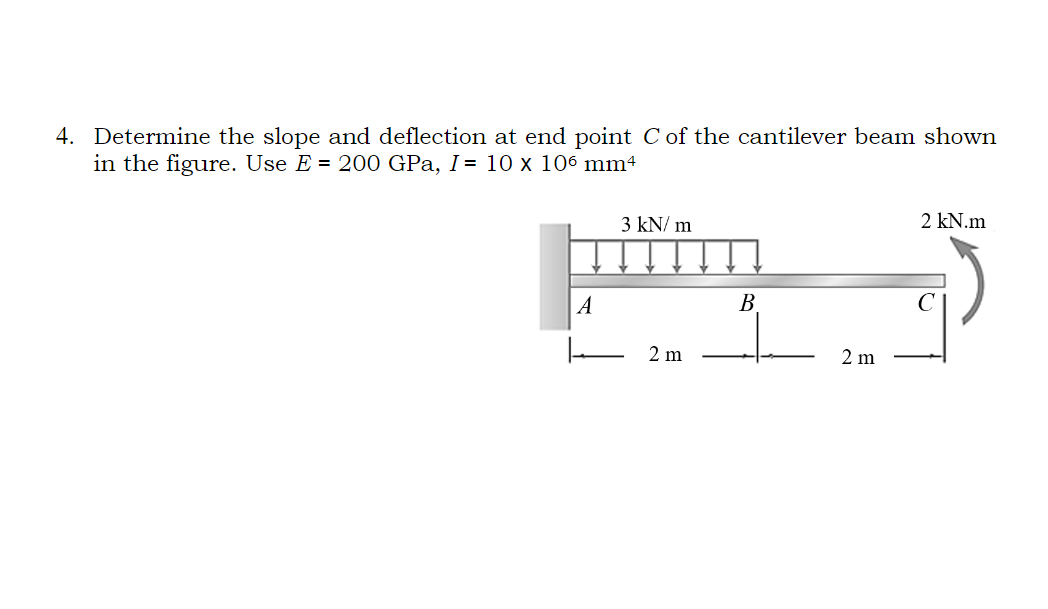4. Determine the slope and deflection at end point C of the cantilever beam shown in the figure. Use E = 200 GPa, I = 10 x 106 mm 3 kN/m 2 kN.m A B 2 m 2 m

• ### For the cantilever beam and loading shown, determine the slope and deflection at point B. Use...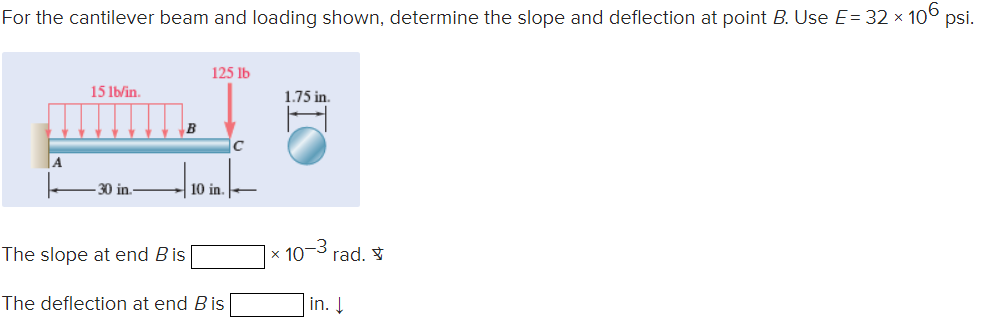For the cantilever beam and loading shown, determine the slope and deflection at point B. Use E = 32 x 106 psi. 125 lb 15 lb/in 1.75 in B 30 in 10 in. The slope at end Bis x 107 rad. The deflection at end B is in.

• ### 2. For the cantilever beam and loading 165 GPa. Shown, calculate the maximum deflection of the...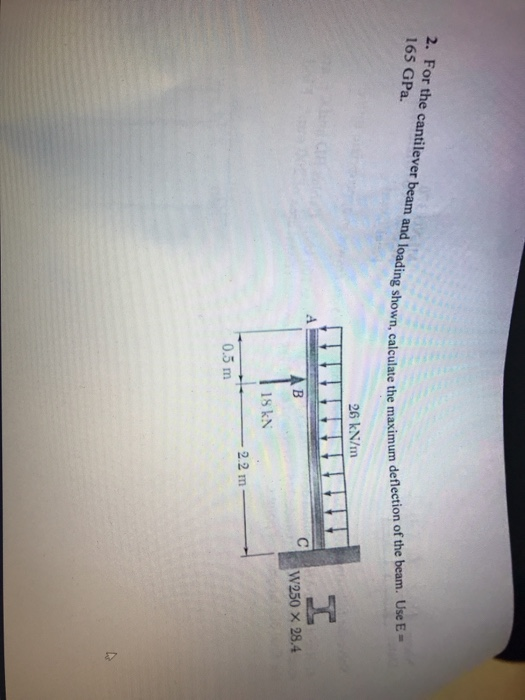2. For the cantilever beam and loading 165 GPa. Shown, calculate the maximum deflection of the beam. Use E- cal 26 kN/m W250 x 28.4 s kN 2.2 m 0.5 m

• ### For the cantilever beam and loading shown, use the method of superposition to determine (a) the...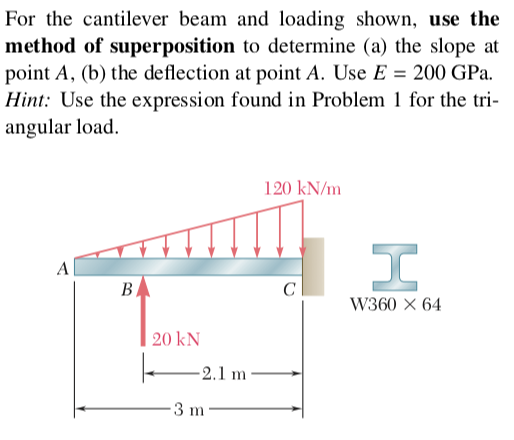For the cantilever beam and loading shown, use the method of superposition to determine (a) the slope at point A, (b) the deflection at point A. Use E 200 GPa. Hint: Use the expression found in Problem 1 for the tri angular load. 120 kN/m W360 × 64 20 kN 2.1 m

• ### 2. For the beam and loading shown, determine the slope and deflection at point B. Where:...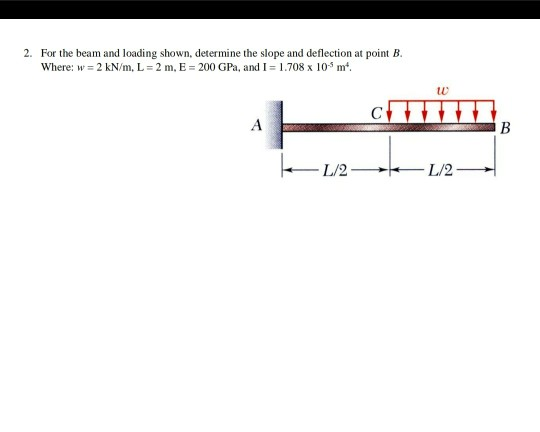2. For the beam and loading shown, determine the slope and deflection at point B. Where: w = 2 kN/m, L = 2 m, E = 200 GPa, and I = 1.708 x 10 m. B 1/2- 1/2

• ### 8. The cantilever beam in Figure Q8 subjects to concentrated loading. The cross section geometry gives...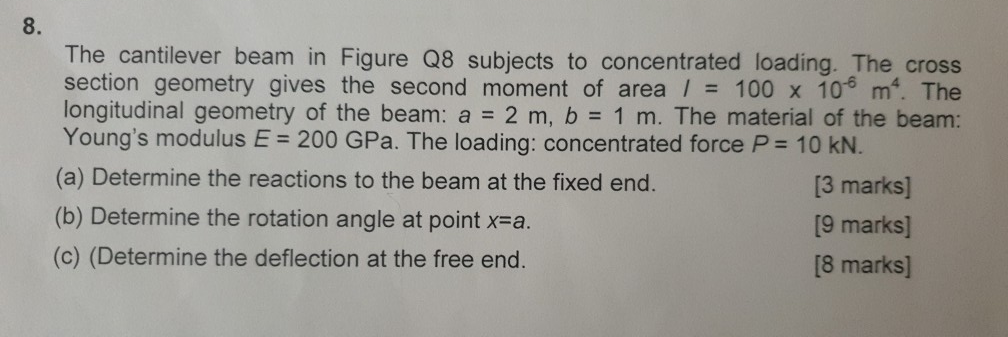8. The cantilever beam in Figure Q8 subjects to concentrated loading. The cross section geometry gives the second moment of area / 100 x 10 m. The longitudinal geometry of the beam: a 2 m, b 1 m. The material of the beam: Young's modulus E 200 GPa. The loading: concentrated force P 10 KN. (a) Determine the reactions to the beam at the fixed end. (b) Determine the rotation angle at point x-a (c) (Determine the deflection at the...